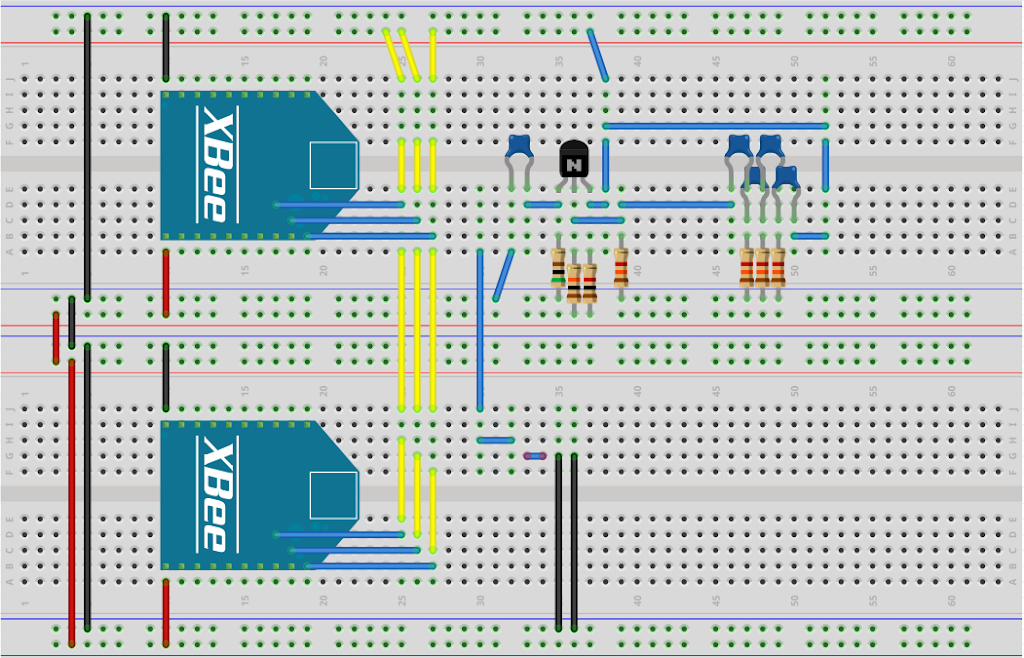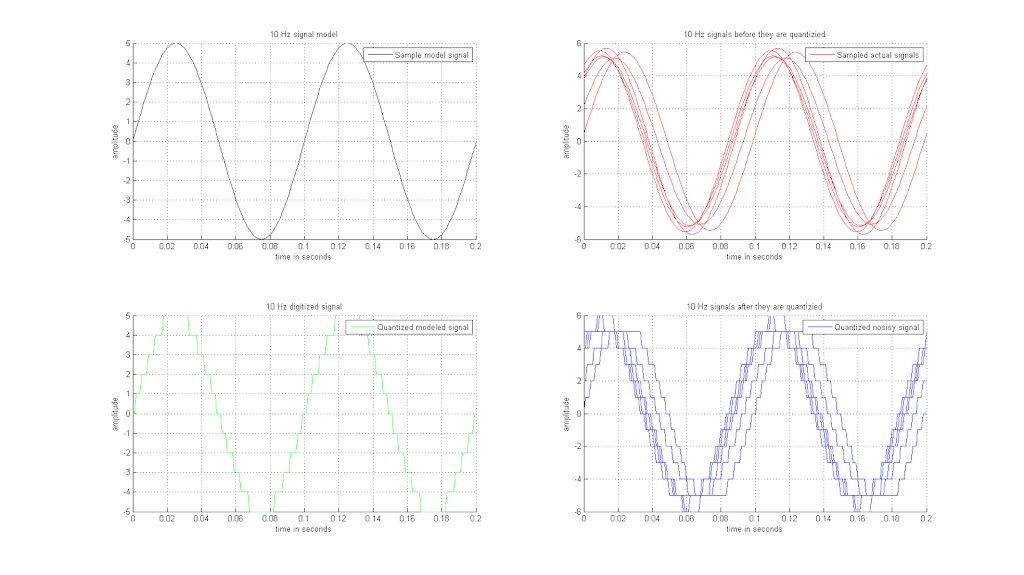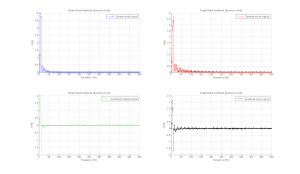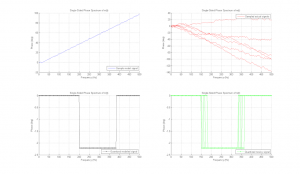Posted on

## Fun with XBees and Jitter 2 of 4: SimulationIntroduction:
Now that we think we know what will happen when we sample our output lets build it!  I need a way to generate the signal for the Xbee to measure. While I have a function generator I realized these may not be common or they may be to expensive so I included the methodology to design a function with a circuit below. But first the high level breadboard design.

I made this diagram using a really cool program from fritzing. Below are two Series 2 Xbees (from Digi) with the first 3 ports of each Xbee connected to the analog signal output [D0:2] for 6 samples total. The red wires are power (5V) and the black wires are ground. The other colors represent signals. The resistors are the correct colors, thanks to fritzing! This design is pretty basic but it is intended to be this way. Ideally it will give enough simplicity to do a robust analysis and also show some utility.
The Xbee’s quantize these signals then transmit them across the mesh. Can these signals be synchronized and reconstructed synthetically? Does a mesh architecture lend efficacy to the reconstruction of a signal? Was Nyquist right? And in doing so will be gain more bandwidth from these XBees? What is bandwidth anyways…and why do we need it so badly? Maybe… 🙂 Well see in future blog posts.Figure 1: The transmitter bread board. This circuit will  first convert an analog signal  generated by the BJT Phase Shift Oscillator to a digital signal then transmit the signal to the receiver board. In this case it is an FEZ Cerbuino from GHI with another series 2 Xbee and serial port attached.

XBee Series 2 Configuration:
I configured the XBee series 2 modules to be in API mode and act as routers. I decided to choose routers rather than endpoints so they could communicate to each other as well as the coordinator. To set up the XBees I used Digi’s tool: XCTU, it is free and works really well for with the XBees. Both Xbees are configured exactly the same by loading the same profile into both of them.

BJT Phase shift Oscillator:
Here is a inexpensive and easy way to build a function generator the function this circuit generates is:
[m(t)=(5+epsilon (t))sinleft(2pi 887+phi (t))]

Schematic:
I used EasyEDA to design this circuit. It is a really nice tool for simple and quick circuits and PCB layout as well as a potential PCB supply source. Well worth the price. Here is my design:

PCB:

Analysis:

The first step is to break this cirucit down into two sub-circuits. The frequency attenuation circuit and the amplifier (BJT) circuit.Next we need to make a few assumptions. For the BJT I’m going to use the Fairchild datasheet. Here are my assumptions and givens:
• Current gain: >= 100 @ 150mA. Because the voltage is lower than the spec sheet recommends the gain is probably closer to 35.
• Power supply voltage: 5V
• Disapation power of 50mW
• Desire a 1 kHz output.
Known relationships:
[R_c_e = R_c + R_e] [P_c_e = P_R_c + P_R_e] [V_c_e = V_R_c + V_R_e] [I_R_c = I_Q_c_e = I_R_c]
First order calculations:

• We need to figure out what this transistor is doing. It has voltage and current which multiply to give power. The transistor will consume half the power. However current will stay the same creating a voltage drop of half across the transistor:
[P =IV_c_e] [I = P_Q_1/V_c_e] [I = 50mW/2.5V = 20mA]
• Next we need to estimate Rc and Re. This will give the max power allowed. These values are not equal in the schematic but to start we make them equal: [R_c=R_e]. If they are equal then the voltage drop across them is half of [V_c_e = 2.5V] so [V_R_e = 1.25V]
• [R_c=R_e=((1/2)V_c_e)/I] [=1.25V/20mA = 625 Omega] [I_c_(_m_a_x_) = V_c_c/(R_c+R_e) = 5V/(625 Omega + 625 Omega) = 40mA ]

• The schematic above shows [R_c = 1.5k Omega] and [R_e = 1k Omega]. So [R_c+R_e = 1.5k Omega] which is pretty close to [1250 Omega] that we calculated above.
• This completes the first subcircuit. The next subcircuit is the attenuation circuit.
• We know we want an oscillation of 1kHz and we can do this by changing the phase of the input signal and sending the positive feedback to the output of the oscillator.  We also know that the output frequency of a 3 stage phase shift oscillator is: [f=1/(2pisqrt{6}RC)]
• . But this is a 4 stage phase shift oscillator. So that changes the equation a bit. I’m going to choose a 1k resistor for the R value since there are two unknowns I thought I would choose a common component. So here’s how it all breaks down:
• [f=1/(2pisqrt{10/7}RC)] [C=1/(2pisqrt{10/7}Rf)]
[C=1/(2pisqrt{10/7}(1kOmega)(1kHz))=0.133 mu F]
• The schematic above shows C as .15uF which is also pretty close to the calculated value above.
• Per the schematic the actual output frequency will be
• [f=1/(2pisqrt{10/7}(1kOmega)(0.15muF)) =887Hz]
• We have two more components to estimate: the emitter capacitor and the base voltage divider resistors.
• We’ll start with the base resistor, [R_b] . Here’s how we get there
• [V_q_t = (V_m_a_x – V_m_i_n)/2 = ((5V – 2.25V)/2)+2.25V = 3.625V]
[V_b = V_R_e + 0.7V = 1.25V + 0.7V = 1.95V]
[V_R_b = V_c_c-V_b = 5V-(1.25V + 0.7V) = 3.05V]
[I_b  = V_b/R_R_c = 1.95V/1kOmega = 1.95mA]
[R_b = V_R_b/I_b = 3.05V/1.95mA = 1.5kOmega]
• Last we need to figure out the emitter capacitor, [C_e]. The purpose of this guy is to keep the gain high enough for the circuit to keep oscillating. The schematic shows 10u lets see if that is even close. Here’s how we get there:
• First I guess at the AC gain since I don’t have the time or equipment to measure it. Probably around 75…this means
[X_C_e = R_e / 75 = 625 / 75 = 8.0Omega]
[C_e = 1 / (2pi f C)X_C_e]
[C_e = 1 / (2 pi (887Hz) (8.03Omega)0.01) = 22 mu F]
…not bad 🙂 but since we guessed at the AC gain we can probably work backwards to get the calculated AC Gain.
[C_e = 10 mu F = 1 / (2 pi f  X_C_e) = 1 / (2 pi (887Hz) (625Omega / [AC gain]))]
then solving for AC gain shows:
[AC gain = C_e2 pi f R_e C_e = 2 pi (887Hz )(625Omega)10 mu F = 35]
What this does is give a bit faster start up time and oscillation closer to the 887Hz. I’ll detail all these differences in a later blog post. They are not really relevant here.

All values in the schematic are calculated to the first order now. There are many more aspects of this circuit but we’ll keep it as simple as possible here.

Netlist:

The Phase-Shift Oscillator 2

.param pi = 3.141593

.func LIMIT(x, y, z) {min(max(x, min(y, z)), max(z, y))}

.func PWR(x,a) {abs(x) ** a}

.func PWRS(x,a) {sgn(x) * PWR(x,a)}

.func stp(x) {u(x)}

V1 RB_2 GND  5

RE GND RE_2  1000

RC RC_1 RB_2  1.500k

RB RB_1 RB_2  1.33k

R7 GND R7_2  1k

R5 GND R5_2  1k

R4 GND VOLPROBE1  10k

R2 GND RB_1  1k

R1 GND R1_2  1k

Q1 RC_1 RB_1 RE_2 2N2222

CE GND RE_2  10u

C7 RC_1 VOLPROBE1  10u

C6 RC_1 R5_2  .15u

C3 R5_2 R1_2  .15u

C2 R7_2 RB_1  .15u

C1 R1_2 R7_2  .15u

* model for a 2n2222 transistor

.model 2n2222 npn (is=19f bf=150 vaf=100 ikf=0.18 ise=50p

+ ne=2.5 br=7.5 var=6.4 ikr=12m isc=8.7p nc=1.2 rb=50 re=0.4

+ rc=0.3 cje=26p tf=0.5n cjc=11p tr=7n xtb=1.5 kf=0.032f af=1)

.control

probe V(VOLPROBE1)

quit

.endc

.END

BOM:
Here here is the list of the types of lower level materials required for this design. I don’t have specific part numbers but they are all available at Digikey or a similar supplier.

Conclusion:
If you made it this far: thanks! Please let me know what you think about this analysis. It is one of the more complete ones I’ve done but I hope it is correct and complete. To iterate what I said at the intro for the what’s i’ll talk about next: The Xbee’s quantize these signals then transmit them across the mesh. Can these signals be synchronized and reconstructed synthetically? Does a mesh architecture lend efficacy to the reconstruction of a signal? Was Nyquist right? And in doing so will be gain more bandwith from these XBees? What is bandwidth anyways…and why do we need it so badly? Maybe… 🙂 Well see in future blog posts.

Posted on

## Fun with XBees and Jitter 1 of 4: TheoryI was thinking about taking a closer look at what phase noise is but then we got side tracked on making a really interesting and convoluted way to measure a signal. In case you are wondering who ‘we’ is. It is me and the frog in my pocket; Jitter is his name. He is a Tylers’ Tree FrogFirst let’s look at the signal we want to measure. Very generally it is this signal:
[m(t)=gleft(T(t)+h(t)+tau (t)right)+epsilon (t)]

but that is a bit to general for this post. So more specifically it is this signal:
[m(t)=sinleft(2pi fF_s)]

Jitter, the frog, reminded me that this is only a model and there are many different types of noise we will be measuring as well. Because of this it is a good idea to expand the model to account for these different types of noise [m(t)=(V_o(t)+epsilon (t))*sinleft(2pi fF_s+phi (t))]

I used this reference for the equation above:
 J. Rutman and F. L. Walls, “Characterization of Frequency Stability in Precision Frequency Sources,” Proceedings of the IEEE, vol. 79, no. 6, pp. 952-960, 1991.

Now we are going to take one of these signals at 10 Hz and send it to 3 ADC inputs on 2 XBee Transceivers.  To do this we need to know a bit about the XBee ADC qualitiesBut here is an overview:

XBee Sample rate

the maximum sample rate that can be achieved while using one A/D is 1 sample/ms or 1KHz. The sample rate can be set with the ATIR command and is set in units of ms, so ATIR=0xA is a sample rate of 10ms or 100Hz.
XBee uses a 10bit A/D converter each sample uses two bytes.

Here are some graphs of what this looks like in theory, using the maximum sample rate.Figure 1: Above are 4 plots describing the model of the signal the XBee will transmit to my computer.  The two graphs on the left the ideal input of the signal. The two graphs on the right are more closely matched to what I expect to be the actual plots. The top two plots are the analog input signals and the bottom two plots are the result of the quantization from the 10-bit ADC converter on board the XBee.

The time domain signals are interesting but there is more to be revealed about these signals. This information is contained in the frequency domain.The general equation to transform the signals from time domain to frequency domain is:
[Fleft(kright)= {mathcal F}left[mleft(tright)right]left(kright) = int^{infty }_{-infty }{left(gleft(Tleft(tright)+hleft(tright)+tauleft(tright)right)+epsilonleft(tright)right)e^{-2pi kt}dt]
The specific case is:

[= }int^{infty }_{-infty }{left({(V}_oleft(tright)+epsilon(t)){sin left(2pi fF_s+phi(t)right) }right)e^{-2pi kt}dt]

These equations can be represented visually as shown below.Figure 2: Above are 4 plots describing the single sided spectrum model of the signal the XBee will transmit to my computer.  The two graphs on the left show the ideal input for single sided spectrum of the signal. The two graphs on the right are more closely matched to what I expect to be the actual plots of the fourier transform. The top two plots are the analog input signals and the bottom two plots are the result of the quantization from the 10-bit ADC converter on board the XBee.Figure 3: Above are 4 plots describing the phase model of the signal the XBee will transmit to my computer.  The two graphs on the left the ideal input of the signal phase. The two graphs on the right are more closely matched to what I expect to be the actual plots of the signal phase. The top two plots are the analog input signals and the bottom two plots are the result of the quantization from the 10-bit ADC converter on board the XBee.